2011-11-01:

## 32 colors

perihelion:gfx:code for fun
Recently I've stumbled on a review of a 1993 Amiga RPG game called Perihelion. I've never played this game (which I've heard is pretty good btw), but after looking at the screenshots I was amazed by what the authors could do with a 32-color limit - they created their palette out of two gradients: a gray one and an orange one. The effect is in my opinion awesome (screenshots below) - actually it's so cool that I've wrote a small program that converts a given image to exactly this 32-color palette (screenshots + source + win32 binary below as well) ;>

## Perihelion

A post about the game (by the game's author if I understand correctly) + the game itself can be found here: click.

And now... three random screenshots (source: page linked above):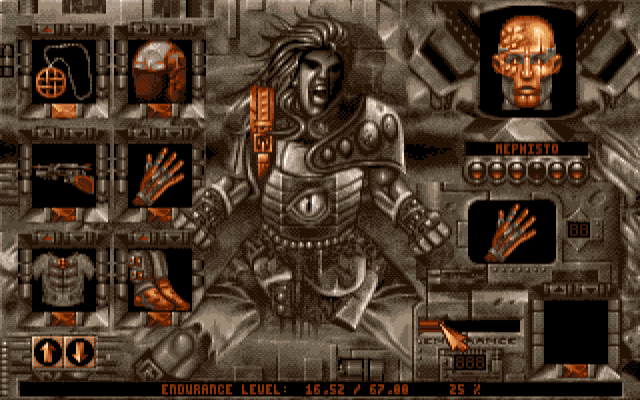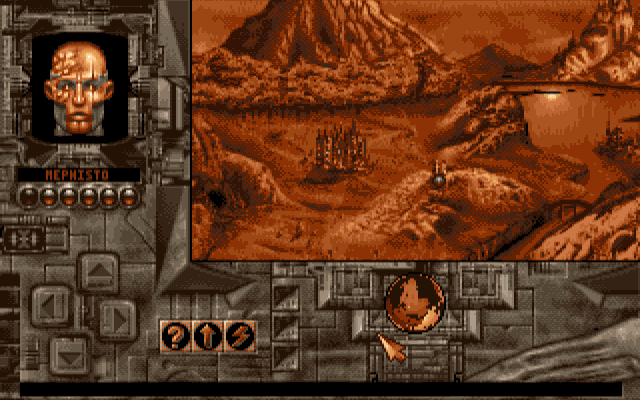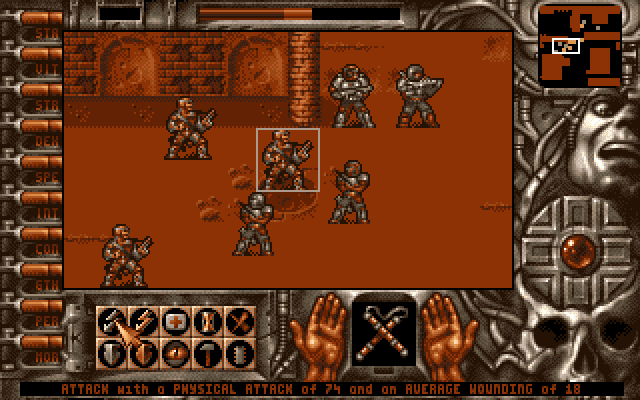## 32colors.cpp

As I've written at the beginning of the post, I really like the effect achieved using such approach to the color palette. So, I've created a converter, that takes any* image and converts it to the bi-gradient 32-color palette with additional dithering.

* by "any" I actually mean a 24bpp PNG or JPG with width being a multiply of 4 - since I've coded this for fun I made some assumptions about the input bitmaps (and decided it's better to write this explanation than actually code it properly ;p).

Anyway, the converter works like this:

- for each pixel
-- calculate the distance between the given pixels color and orange
-- if the distance is less or equal A use the orange palette
-- else use the gray palette
-- calculate the lightness of the pixels color
-- add a dithering value to the lightness
-- decrease the lightness depth to 4 bits (16 possible values)
-- "multiply" the lightness with the selected palette
-- and put that as the new pixel

As far as the dithering goes, I've used Bayer' (it's that sentiment I have to the "good old times" when Windows wasn't 24+ bits by default and used this dithering). Unavowed suggested that I used Stuckie' dithering, but for random reasons I stayed with Bayer.

Calculating the distance between colors is an interesting problem - just how would you calculate some value that tells you about how much two colors differ from each other? Well, I guess it's not a problem of "how" to do it (because I'm pretty sure everyone can think of at least a couple of ways), but which method to choose.
My idea was to treat both RGB colors as a 3D vector/point, normalize them (i.e. divide each value by the length of the vector), and calculate a 3D distance between the normalized points.
Arashi on the other hand suggested an idea to derive the hue from RGB and use the angular distance between the hues as the distance (expressed in degrees).
In the end I've implemented both ideas since they give different results.

As far as A goes, it's a parameter expressed either in the distance units between normalized vectors (that would be from 0.0 to give or take 1.0) or in degrees (0 to 359) in the other.

The code + a Windows binary can be downloaded here: 32colors.zip (392kb; src+win32 bin).
Of course you should remember that this was coded for fun and has some bugs ;>

And now... a couple of screen shots (you can click-to-zoom the right side ones).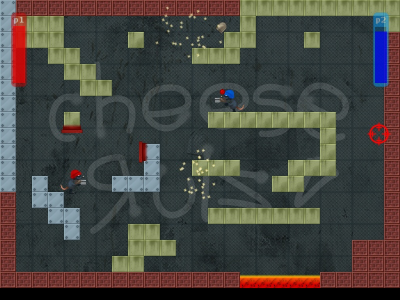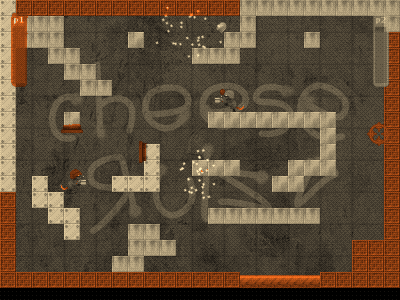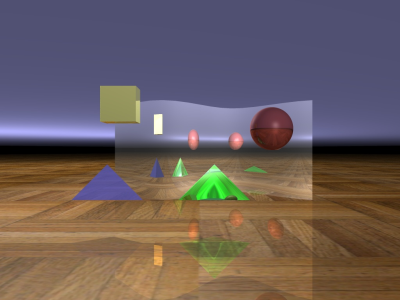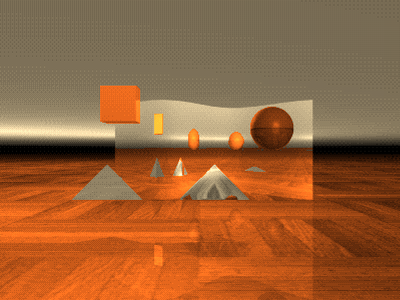An as a bonus: a converted photo by Arashi and .S.K.Y. (using a tone of blue instead of orange):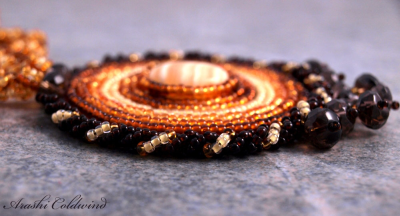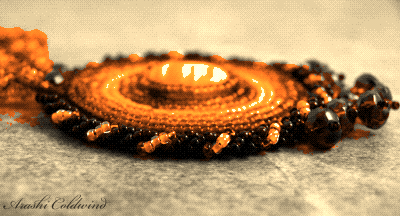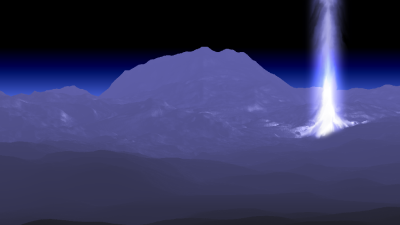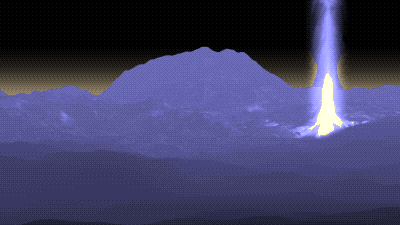And that's that.